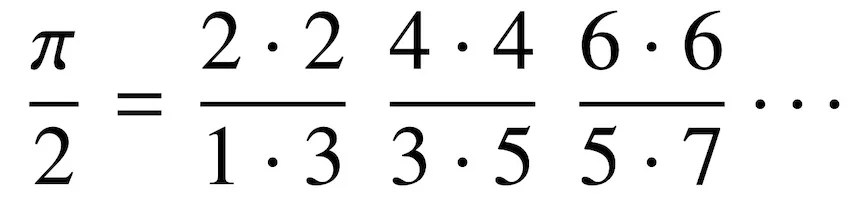# Researchers Discovered A Unique Formula Of Pi Hidden In Hydrogen Atoms

• Physicists accidentally found that classic formula of pi derived in the 17th century characterizes the hydrogen atom.
• Their derivation connects pure math with quantum physics.

Originally defined as the ratio of the circumference of any circle to the diameter of that circle, pi has now numerous equivalent definitions and appears in a wide range of formulas in all fields of mathematics and physics.

In 1655, an English clergyman and mathematician John Wallis published a treatise describing a formula for pi as an infinite product of ratios. Since infinitesimal calculus didn’t exist then, it was very difficult to analyze the convergence issues of the formula. But later, fundamental theorems of algebra and infinite product confirmed the ‘Wallis product’.Formula for pi as the infinite product

In 2015, researchers at the University of Rochester discovered the same classic formula can be derived from a variational computation of the hydrogen atom spectrum. The fact that a pure mathematical formula derived in 1655 denotes the structure of element that was discovered three centuries later, is absolutely fascinating.

### Connecting Pure Math With Quantum Physics

The discovery was made when a particle physicist Carl Hagen was giving a lecture on quantum mechanics: he was elaborating how to calculate the energy state of a hydrogen atom using a quantum mechanical method called variation principle.

In quantum physics, the variational technique can be applied to molecules to approximate their energy states. Usually, the technique used for large and complex molecules where finding the exact energy states isn’t possible.

Reference: American Institute of Physics | doi:10.1063/1.4930800

Since hydrogen is the simplest element with only one electron in its orbit, its energy level can be calculated precisely. Hagen used variational principle on this element and then compared outcomes to the exact solutions, to measure the estimated error. This is where he found something unusual.

The error in the approximation was 15% for the ground state and 10% for the first excited state. As the excited states expanded, the error got smaller. This was quite surprising because variational solution only yields higher errors for the lowest energy levels.

The research team dug deeper and discovered that the variation principle limit approaches the hydrogen model discovered in 1913 by physicist Neil Bohr, which shows that electrons’ orbits are perfectly circular.Moreover, the correspondence principle tells that for bigger radius orbits, the quantum systems’ behavior can be well-explained by classical physics. The electron path is quite fuzzy at the lower energy orbits. As you move to more excited states, the orbits get more sharply defined and inconsistencies in the radius reduce.

From the variation principle limit as the energy-level elevated, the research team was able to extract the ‘Wallis product’ for pi. This new derivation connects pure math with quantum physics.Written by
###### Varun Kumar

Varun Kumar is an experienced science and technology journalist interested in machines, AI, and space exploration. He received a Master's degree in computer science from Indraprastha University. To find out what his latest project is, feel free to directly email him at [email protected]